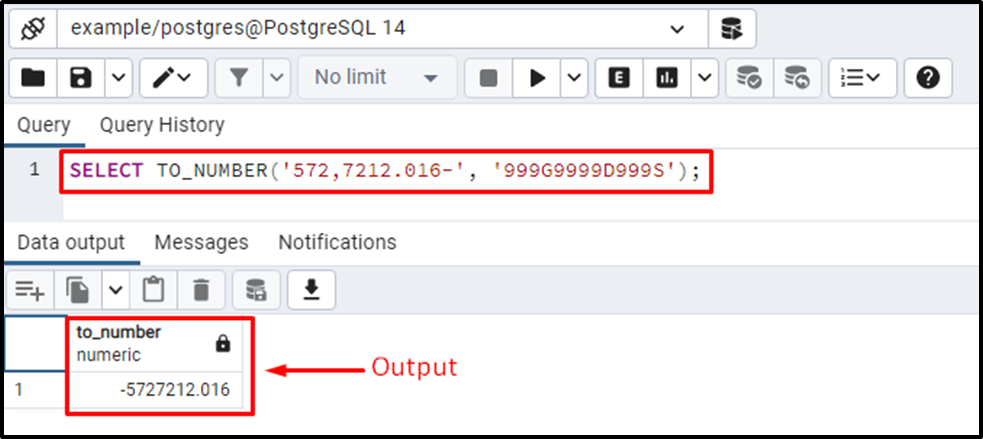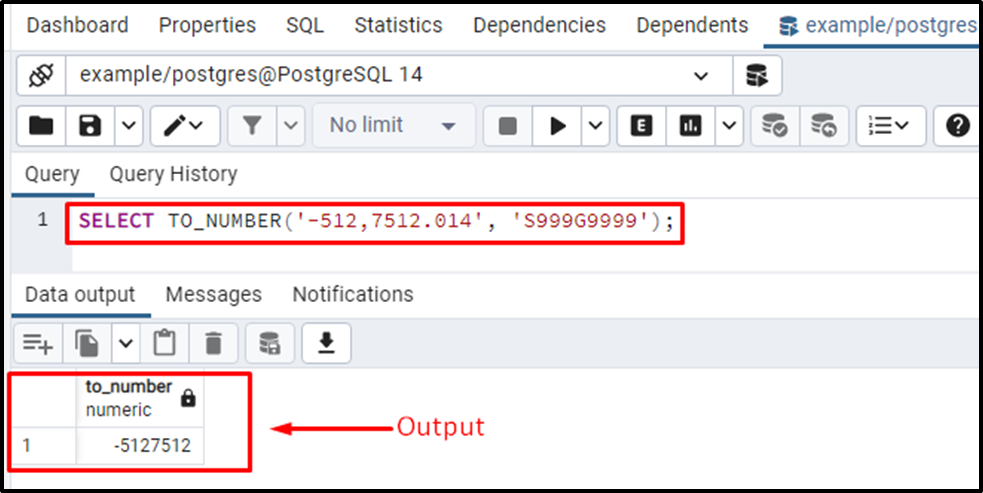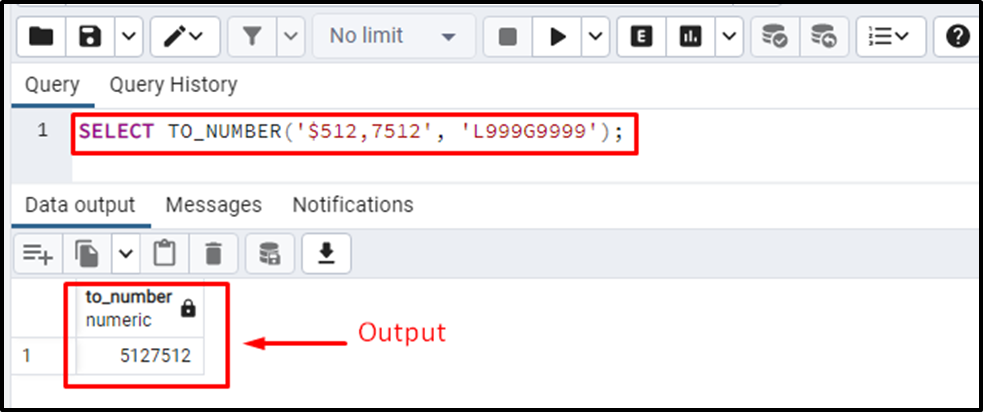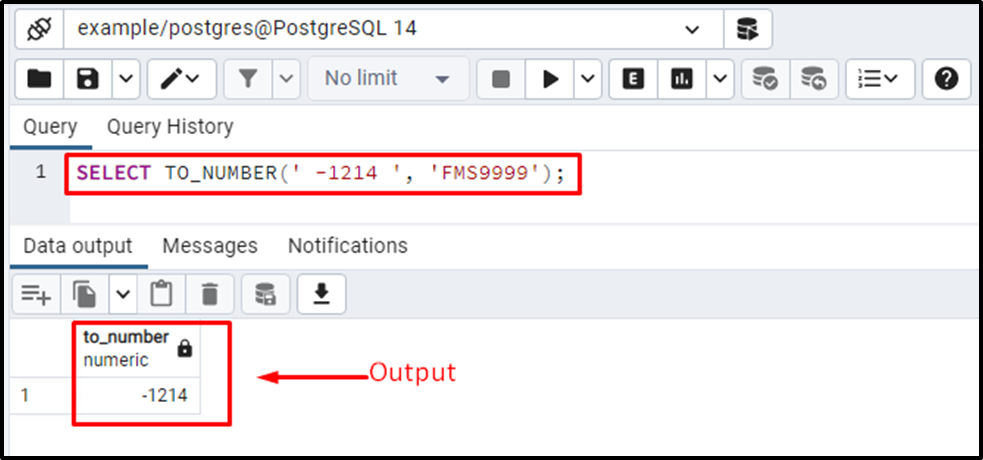##### How to Use TO_NUMBER() Function in PostgreSQL

In Postgres, TO_NUMBER() is a built-in function that converts a string/text into a number. The TO_NUMBER() function accepts two parameters, i.e., the first parameter is a “string” that needs to be converted. While the second parameter represents a “format” based on which the targeted string will be converted.

This write-up will explain the working of the TO_NUMBER function with the help of different examples. So, let’s start.

How to Use the TO_NUMBER() in PostgreSQL?

The basic syntax of the TO_NUMBER() function will be as follows:

`TO_NUMBER(str, format);`

Here, TO_NUMBER() is a built-in Postgres Function that requires the following parameters:

- “str” represents a string/text to be converted.

- The second parameter must be a valid format. The targeted string/text will be converted into a number according to the given format.

Here is the list of valid formats for the TO_NUMBER() function:

- “.” (dot/period) represents a decimal.

- D represents a decimal that utilizes a locale.

- 0 represents a number with leading 0’s.

- 9 represents one digit.

- Comma “,” represents a group separator.

- G represents a group separator that utilizes locale.

- RN represents a roman value between the range ‘1-3999’.

- FM(Fill mode) deletes the leading/trailing spaces.

- S represents a sign.

- SG represents the plus/minus sign.

- PL represents a plus sign for numbers greater than 0(positive numbers).

- MI represents a minus sign for the numbers less than 0(negative numbers).

- PR represents a negative value within angle brackets.

- L represents a currency symbol.

- TH/th represents the ordinal number suffix.

Now, let’s understand the working of the TO_NUMBER() function by implementing it practically.

Example #1: How to Use the TO_NUMBER Function in Postgres?

Suppose we have a string “572,7212.016-”. Let’s convert the given string into a numeric value using the following statement:

`SELECT TO_NUMBER('572,7212.016-', '999G9999D999S');`

In the above example, “573,7212.016-” is a string that needs to be converted into a number. We utilized the “999G9999D999S” format to convert the user-specified string into a number. The stepwise description of the specified format is as follows:

- Three 9’s at the start represent that there are three digits at the start of the string.

- G at the fourth position represents that three will be a group separator “,” at the fourth position.

- The four 9’s after the G symbol represent that there are four more digits after the group separator.

- D after four 9’s represents that there will be a decimal point after four digits.

- Three 9’s after the D represent that there will be three digits after the fractional part.

- S at the end represents a sign. Since we have a negative number, we will get a minus sign.The output clarified that the TO_NUMBER function successfully converted the given string into a number.

Example #2: How to Convert a String into an Integer/Numeric Value(Skip the Floating Points)?

Suppose we have to convert a string(“572,7212.016-”) into a number, but we don’t need the decimal part of the given string:

`SELECT TO_NUMBER('-512,7512.014', 'S999G9999');`

In this example, the TO_NUMBER() function will convert the given string (i.e., 572,7212.016-) into a number. Since we didn’t specify the D symbol and 9 in place of decimal/period and digits that come after the period. Therefore, the TO_NUMBER() function will return only the integral part and skip the fractional part.The output authenticates the working of the TO_NUMBER() function as it succeeded in converting the given string into a number.

Example #3: How to Convert a String(Cash Amount) into a Number?

Consider a string containing a special symbol (i.e., a currency symbol). Let’s utilize the TO_NUMBER() function to convert any string into a number:

`SELECT TO_NUMBER('\$512,7512', 'L999G9999');`

In the TO_NUMBER() function, the L symbol represents a currency symbol.From the output, it is clear that the TO_NUMBER() function succeeded in converting the given string to a number.

Example #4: How to Convert a String(Containing Leading and Trailing Spaces) into a Number?

In the TO_NUMBER() function, the FM symbol is used to trim the leading and trailing spaces from a string. Let’s convert a string type value ‘ -1214 ' into a number type value:

`SELECT TO_NUMBER(' -1214 ', 'FMS9999');`

In the format parameter, we specified the FM at the start that will skip the leading and trailing spaces. Next, we utilized the S symbol to specify a symbol, and at the end, four 9’s represent that there will be four digits after the (minus) sign.The output proves the working of the TO_NUMBER() function.

Conclusion

TO_NUMBER() is a built-in Postgres function that converts a string/text into a number. The TO_NUMBER() function accepts two parameters: the first one represents a “string” that needs to be converted. While the “format” parameter specifies the conversion criteria for the given string. There are multiple valid formats for the TO_NUMBER() function whose usage depends on the situation. This write-up explained how the TO_NUMBER() function works in PostgreSQL with the help of several examples.